1. /
2. UK Board
3. /
4. Class 12
5. /
6. Physics
7. /
8. NCERT Solutions For Class...

# NCERT Solutions For Class 11 physics Kinetic Theory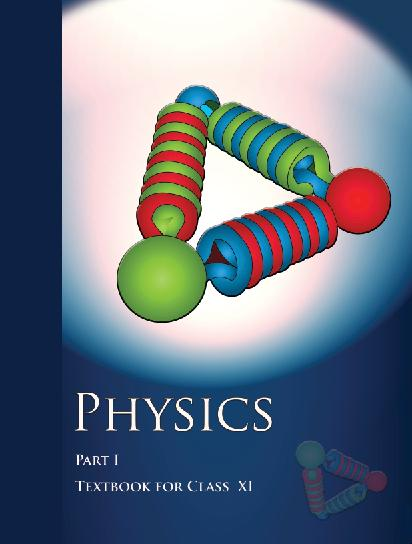## NCERT Class 11 Physics Chapter wise Solutions

• 1 – Physical World
• 2 – Units and Measurements
• 3 – Motion in a Straight line
• 4 – Motion in a Plane
• 5 – Laws of Motion
• 6 – Work, Energy and Power
• 7 – System of Particles and Rotational motion
• 8 – Gravitation
• 9 – Mechanical Properties of Solids
• 10 – Mechanical properties of fluids
• 11 – Thermal Properties of matter
• 12 – Thermodynamics
• 13 – Kinetic Theory
• 14 – Oscillations
• 15 – Waves

## CHAPTER 13 KINETIC THEORY

• 13.1 Introduction
• 13.2 Molecular nature of matter
• 13.3 Behaviour of gases
• 13.4 Kinetic theory of an ideal gas
• 13.5 Law of equipartition of energy
• 13.6 Specific heat capacity
• 13.7 Mean free path

## NCERT Solutions For Class 11 physics Kinetic Theory

1. Estimate the fraction of molecular volume to the actual volume occupied by oxygen gas at STP. Take the diameter of an oxygen molecule to be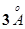.

2. Molar volume is the volume occupied by 1 mol of any (ideal) gas at standard temperature and pressure (STP: 1 atmospheric pressure, 0 °C). Show that it is 22.4 liters.

###### 3. Figure 13.8 shows plot of PV/T versus Poor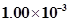kg of oxygen gas at two different temperatures.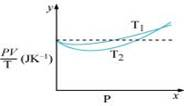(a) What does the dotted plot signify?

(b) Which is true: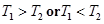?

(c) What is the value of PV/T where the curves meet on the y-axis?

(d) If we obtained similar plots forkg of hydrogen, would we get the same value of PV/T at the point where the curves meet on the y-axis? If not, what mass of hydrogen yields the same value of PV/T (for low pressure high temperature region of the plot)? (Molecular mass of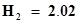u, of O2= 32.0 u, R =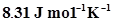.)

4. An oxygen cylinder of volume 30 litres has an initial gauge pressure of 15 atm and a temperature of 27 °C. After some oxygen is withdrawn from the cylinder, the gauge pressure drops to 11 atm and its temperature drops to 17 °C. Estimate the mass of oxygen taken out of the cylinder (R = 8.31 J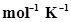, molecular mass of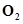= 32 u).

5. An air bubble of volume 1.0 cm3rises from the bottom of a lake 40 m deep at a temperature of 12 °C. To what volume does it grow when it reaches the surface, which is at a temperature of 35 °C?

6. Estimate the total number of air molecules (inclusive of oxygen, nitrogen, water vapour and other constituents) in a room of capacity 25.0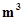at a temperature of 27 °C and 1 atm pressure.

7. Estimate the average thermal energy of a helium atom at (i) room temperature (27 °C), (ii) the temperature on the surface of the Sun (6000 K), (iii) the temperature of 10 million Kelvin (the typical core temperature in the case of a star).

8. Three vessels of equal capacity have gases at the same temperature and pressure. The first vessel contains neon (monatomic), the second contains chlorine (diatomic), and the third contains uranium hexafluoride (polyatomic). Do the vessels contain equal number of respective molecules? Is the root mean square speed of molecules the same in the three cases? If not, in which case is the largest?

9. At what temperature is the root mean square speed of an atom in an argon gas cylinder equal to the rms speed of a helium gas atom at – 20 °C? (atomic mass of Ar = 39.9 u, of He = 4.0 u).

10. Estimate the mean free path and collision frequency of a nitrogen molecule in a cylinder containing nitrogen at 2.0 atm and temperature 17 °C. Take the radius of a nitrogen molecule to be roughly 1.0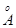. Compare the collision time with the time the molecule moves freely between two successive collisions (Molecular mass of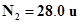).

11. A metre long narrow bore held horizontally (and closed at one end) contains a 76 cm long mercury thread, which traps a 15 cm column of air. What happens if the tube is held vertically with the open end at the bottom?

12. From a certain apparatus, the diffusion rate of hydrogen has an average value of 28.7 cm3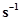. The diffusion of another gas under the same conditions is measured to have an average rate of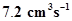. Identify the gas.

[Hint: Use Graham’s law of diffusion: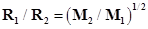, where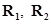are diffusion rates of gases 1 and 2, and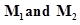their respective molecular masses. The law is a simple consequence of kinetic theory.]

13. A gas in equilibrium has uniform density and pressure throughout its volume. This is strictly true only if there are no external influences. A gas column under gravity, for example, does not have uniform density (and pressure). As you might expect, its density decreases with height. The precise dependence is given by the so-called law of atmospheres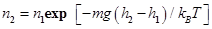Where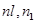refer to number density at heights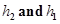respectively. Use this relation to derive the equation for sedimentation equilibrium of a suspension in a liquid column: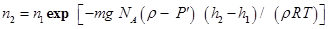Where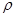is the density of the suspended particle, and‘ that of surrounding medium. [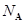is Avogadro’s number, and R the universal gas constant.] [Hint: Use Archimedes principle to find the apparent

14. Given below are densities of some solids and liquids. Give rough estimates of the size of their atoms: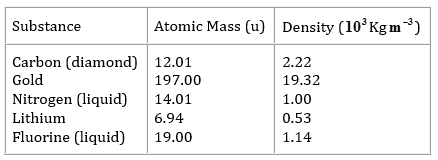[Hint: Assume the atoms to be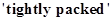in a solid or liquid phase, and use the known value of Avogadro’s number. You should, however, not take the actual numbers you obtain for various atomic sizes too literally. Because of the crudeness of the tight packing approximation, the results only indicate that atomic sizes are in the range of a few].

## NCERT Solutions for Class 11 Physics

NCERT Solutions Class 11 Physics PDF (Download) Free from myCBSEguide app and myCBSEguide website. Ncert solution class 11 physics includes text book solutions from both part 1 and part 2. NCERT Solutions for CBSE Class 11 Physics have total 15 chapters. 11 Physics NCERT Solutions in PDF for free Download on our website. Ncert physics class 11 solutions PDF and physics ncert class 11 PDF solutions with latest modifications and as per the latest CBSE syllabus are only available in myCBSEguide

## CBSE app for Class 11

To download NCERT Solutions for class 11 Physics, Chemistry, Biology, History, Political Science, Economics, Geography, Computer Science, Home Science, Accountancy, Business Studies and Home Science; do check myCBSEguide app or website. myCBSEguide provides sample papers with solution, test papers for chapter-wise practice, NCERT solutions, NCERT Exemplar solutions, quick revision notes for ready reference, CBSE guess papers and CBSE important question papers. Sample Paper all are made available through the best app for CBSE students and myCBSEguide website.### Test Generator

Create question paper PDF and online tests with your own name & logo in minutes.### myCBSEguide

Question Bank, Mock Tests, Exam Papers, NCERT Solutions, Sample Papers, Notes